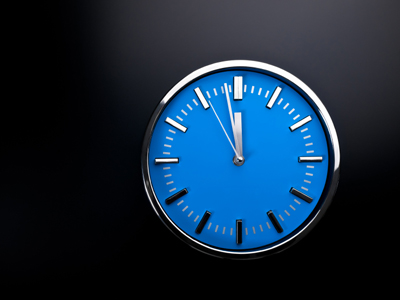Take time to play this quiz all about time!

# Time 6

This Math quiz is called 'Time 6' and it has been written by teachers to help you if you are studying the subject at elementary school. Playing educational quizzes is an enjoyable way to learn if you are in the 3rd, 4th or 5th grade - aged 8 to 11.

It costs only \$12.50 per month to play this quiz and over 3,500 others that help you with your school work. You can subscribe on the page at Join Us

This quiz about time is going to look at the 24 hour clock. This clock is used extensively, for example: in computers, in the military and in transport.

Using the 24 hour clock removes all possibility of making a mistake with the time, for example: 'Meet me at 6 o'clock'. Is that 6 o'clock in the morning or in the evening? The use of 'am' and 'pm' doesn't always help because not all people know what they mean: pm = post meridiem = between 12 noon and 12 midnight; am = ante meridiem = between 12 midnight and 12 noon.

Some adults find the 24 hour clock difficult to remember. 16:18 is not as easy as nearly twenty past four, is it? It is a useful thing to learn and you should find it easier than adults. Why? Because you have grown up with computers which always show the time in 24 hours.

Go straight to Quiz

00:00 = 12:00 midnight (start of day)
01:00 = 1:00 a.m.
02:00 = 2:00 a.m.
03:00 = 3:00 a.m.
04:00 = 4:00 a.m.
05:00 = 5:00 a.m.
06:00 = 6:00 a.m.
07:00 = 7:00 a.m.
08:00 = 8:00 a.m.
09:00 = 9:00 a.m.
10:00 = 10:00 a.m.
11:00 = 11:00 a.m.
12:00 = 12:00 (noon)
13:00 = 1:00 p.m.
14:00 = 2:00 p.m.
15:00 = 3:00 p.m.
16:00 = 4:00 p.m.
17:00 = 5:00 p.m.
18:00 = 6:00 p.m.
19:00 = 7:00 p.m.
20:00 = 8:00 p.m.
21:00 = 9:00 p.m.
22:00 = 10:00 p.m.
23:00 = 11:00 p.m.
00:00 = 12:00 midnight (end of day)
1.
Using the 24-hour conversion table, how would the time appear on the 24 hour clock?
6:30 am
18:30
06:30
6:30
8:30
This is read as 'zero six thirty hours'. TIP: Between midnight and noon, you don't have to do any conversion, e.g. 5:40 am = 05:40
2.
Using the 24-hour conversion table, how would the time appear on the 24 hour clock?
7:00 pm
19:00
07:00
7:00
19
This is read as 'nineteen hundred hours'. TIP: After 12 noon until midnight, you add 12 to the hour, e.g. 5:40 pm = 17:40
3.
Using the 24-hour conversion table, how would the time appear on the 24 hour clock?
6:35 am
16:35
6:35
18:35
06:35
This is read as 'zero six thirty-five hours'
4.
Using the 24-hour conversion table, how would the time appear on the 24 hour clock?
9:00 pm
9:00
21
09:00
21:00
This is read as 'twenty-one hundred hours'
5.
Using the 24-hour conversion table, how would the time appear on the 24 hour clock?
11:30 am
11:30
23:30
011:30
22:30
This is read as 'eleven thirty hours'
6.
Using the 24-hour conversion table, how would the time appear on the 24 hour clock?
5:15 pm
17:15
05:15
5:15
07:15
This is read as 'seventeen fifteen hours'
7.
Using the 24-hour conversion table, how would the time appear on the 24 hour clock?
4 pm
04
04:00
16:00
16
This is read as 'sixteen hundred hours'
8.
Using the 24-hour conversion table, how would the time appear on the 24 hour clock?
10:45 pm
22:45
10:45
010:45
23:45
This is read as 'twenty-two forty-five hours'
9.
Using the 24-hour conversion table, how would the time appear on the 24 hour clock?
3:15 am
15:15
03:15
3:15
003:15
This is read as 'zero three hundred fifteen hours'
10.
Using the 24-hour conversion table, how would the time appear on the 24 hour clock?
12:00 midnight
12
0:00
0:0
00:00
This is read as 'zero zero hundred hours'. Note: The day begins at midnight, and the last minute of the day begins at 23:59 and ends at 24:00 BUT this is shown as 00:00. Some people do use 24:00, for example: 'Open from 00:00 to 24:00' - this shows that the business is open for the whole 24 hour period. To use 'Open from 00:00 to 00:00' might be a bit confusing. In general, avoid using 24:00 when telling the time
Author:  Frank Evans